## Example Questions

← Previous 1

### Example Question #1 : How To Factor A Number

Column A

5!/3!

Column B

6!/4!

The quantity in Column A is greater.

The quantity in Column B is greater.

The relationship cannot be determined from the information given.

The two quantities are equal.

The quantity in Column B is greater.

Explanation:

This is a basic factorial question. A factorial is equal to the number times every positive whole number smaller than itself. In Column A, the numerator is 5 * 4 * 3 * 2 * 1 while the denominator is 3 * 2 * 1.

As you can see, the 3 * 2 * 1 can be cancelled out from both the numerator and denominator, leaving only 5 * 4.

The value for Column A is 5 * 4 = 20.

In Column B, the numerator is 6 * 5 * 4 * 3 * 2 * 1 while the denominator is 4 * 3 * 2 * 1. After simplifying, Column B gives a value of 6 * 5, or 30.

Thus, Column B is greater than Column A.

### Example Question #81 : Arithmetic

Which of the following integers are factors of both 24 and 42?

12
5
8
7
3
Explanation:

3 is the only answer that is a factor of both 24 and 42. 42/3 = 14 and 24/3 = 8.  The other answers are either a factor of 24 OR 42 or neither, but not both.

### Example Question #1 : How To Factor A Number

721(413) + 211(721) is equal to which of the following?

413(721 + 211)

211(413 + 721)

721(413 + 211)

(721 + 413)(211 +721)

(721 + 211)(413 + 721)

721(413 + 211)

Explanation:

The answer is 721(413 + 211) because we can pull out a common factor, or 721, from both sides of the equation.

### Example Question #2 : Other Factors / Multiples

n is a positive integer .  p = 4 * 6 * 11 * n

Quantity A: The remainder when p is divided by 5

Quantity B: The remainder when p is divided by 33

Quantity A is greater.

The two quantities are equal.

Quantity B is greater.

The relationship cannot be determined from the information given.

The relationship cannot be determined from the information given.

Explanation:

Let's consider Quantity B first.  What will the remainder be when p is divided by 33?

4, 6 and 11 are factors of p which means that 2 * 2 * 2 * 3 * 11 * n will equal p.  We can group the 3 and 11 to see that 33 will always be a factor of p and will have no remainder. Thus Quantity B will always equal 0 no matter the value of n.

Now consider Quantity A. Let's consider first the values for p when n equals 1 through 5. When n = 1, p = 264, and the remainder is 4/5 or 0.8.

n = 2, p = 528, and the remainder is 3/5 or 0.6.

n = 3, p = 792, and the remainder is 2/5 or 0.4.

n = 4, p = 1056, and the remainder is 1/5 or 0.2.

n = 5, p = 1320, and the remainder is 0 (because when n = 5, 5 becomes a factor of p and thus there is no remainder.

Because Quantity A can be equal to or greater than B, there is not enough information given to determine the relationship.

### Example Question #81 : Arithmetic

Quantitative Comparison

Quantity A: number of 2's in the prime factorization of 32

Quantity B: number of 2's in the prime factorization of 60

The two quantities are equal.

Quantity A is greater.

Quantity B is greater.

The relationship cannot be determined from the information given.

Quantity A is greater.

Explanation:

32 = 2 * 16 = 2 * 4 * 4 = 2 * 2 * 2 * 2 * 2 = 25, so Quantity A = 5.

60 = 2 * 30 = 2 * 6 * 5 = 2 * 2 * 3 * 5 = 22 * 3 * 5, so Quantity B = 2.

Quantity A is greater.  Even though 60 is a larger number than 32, 32 has more 2's in its prime factorization.

### Example Question #1 : How To Factor A Number

Ifis an integer and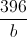is an integer, which of the following could be the value of?Explanation:

Because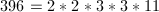, the answer choice that has a factorization set that cancels out completely with 396 will produce an integer. Only 18 fits this qualification, since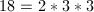.

### Example Question #2 : How To Factor A Number

What is the sum of the individual factors of 100 and 200?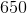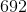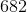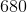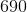Explanation:

Do not try to count out the factors. A neat formula for finding the sum of factors of a number can be utilized by first determining the prime factorization of the number.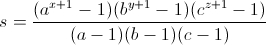where s is the sum, a, b, and c are factors, and x, y, and z are the powers of these factors.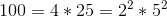Then, a = 2, b = 5, x = 2, y = 2.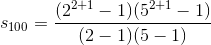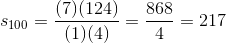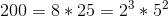Then, a = 2, b = 5, x = 3, y = 2.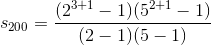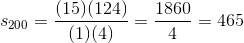Now we can add our two sums.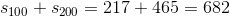### Example Question #1 : How To Factor A Number

What is the largest possible integer value ofif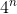divides 16! evenly?Explanation:

This question is really asking, “How many factors of 4 are there in 16!”?  To ascertain this, list all the even numbers and count the total number of 2s among those factors.

Respectively, 16, 14, 12, 10, 8, 6, 4, 2 have 4, 1, 2, 1, 3, 1, 2, 1 factors of 2.

The total then is 15. This means that you have a factor of 215, which is the same as 47 * 2; therefore, since you are asked for the largest integer value of n, 7 is your answer.

Any larger integer value would not allow 4n to divide 16! evenly.

### Example Question #3 : Other Factors / Multiples

If the product of two distinct integer is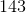, which of the following could not represent the sum of those two integers?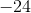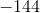Explanation:

Since we're dealing with a product that comes out to a positive value, it could be the product of two positives or two negatives.

That being said, consider the ways we could factor: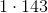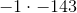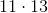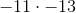For each of these four possible factors, there are four possible sums:### Example Question #4 : Other Factors / Multiples

If the product of two distinct integers is, which of the following could not represent the sum of those two integers?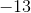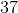Explanation:

When the product of two numbers is positive, that means that either both numbers were positive, or both numbers were negative.

Now, considering the waycould be factored: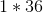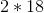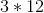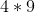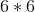And of course the cases where both values are negative. For each of these potential factors, the sums are then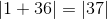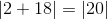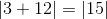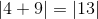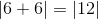Absolute value signs are used to denote that either a sum or it's negative suffices. However, recall that we're told the two integers are distinct!

Due to this, neitheroris an acceptable answers, because both the integers would be equivalent and not distinct.

← Previous 1

### All GRE Math Resources